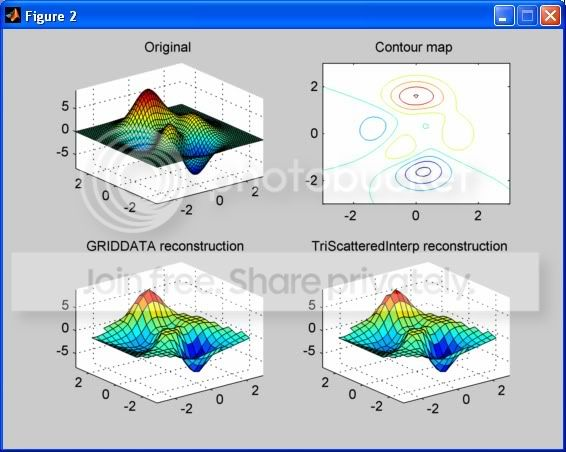Graviton -4 years ago 332
C# Question

# How Do I Generate a 3-D Surface From Isolines?

I have a set of isoline points (or contour points) such as this:

alt text http://www.rcad.eu/triang&isolines%20example1.jpg

Each point on an isoline has its own respective X, Y, and Z coordinate. Since they are isolines, that means that each point will have a unique X-Y pair, but points on the same line will have the same Z coordinate.

Now, is there any algorithm or any software packages (either in C# or C++ or MATLAB) that I can use to interpolate the isoline points into a full 3-D surface?

P/S: I am not just interested in the final output, I am interested in getting the interpolated surface data so that I can plot the surface myself.

Edit: C++ solutions are welcomed as well.gnovice
Answer Source

In MATLAB you can use either the function `griddata` or the `TriScatteredInterp` class (Note: as of R2013a `scatteredInterpolant` is the recommended alternative). Both of these allow you to fit a surface of regularly-spaced data to a set of nonuniformly-spaced points (although it appears `griddata` is no longer recommended in newer MATLAB versions). Here's how you can use each:

• `griddata`:

``````[XI,YI,ZI] = griddata(x,y,z,XI,YI)
``````

where `x,y,z` each represent vectors of the cartesian coordinates for each point (in this case the points on the contour lines). The row vector `XI` and column vector `YI` are the cartesian coordinates at which `griddata` interpolates the values `ZI` of the fitted surface. The new values returned for the matrices `XI,YI` are the same as the result of passing `XI,YI` to `meshgrid` to create a uniform grid of points.

• `TriScatteredInterp` class:

``````[XI,YI] = meshgrid(...);
F = TriScatteredInterp(x(:),y(:),z(:));
ZI = F(XI,YI);
``````

where `x,y,z` again represent vectors of the cartesian coordinates for each point, only this time I've used a colon reshaping operation `(:)` to ensure that each is a column vector (the required format for `TriScatteredInterp`). The interpolant `F` is then evaluated using the matrices `XI,YI` that you must create using `meshgrid`.

## Example & Comparison

Here's some sample code and the resulting figure it generates for reconstructing a surface from contour data using both methods above. The contour data was generated with the `contour` function:

``````% First plot:

subplot(2,2,1);
[X,Y,Z] = peaks;  % Create a surface
surf(X,Y,Z);
axis([-3 3 -3 3 -8 9]);
title('Original');

% Second plot:

subplot(2,2,2);
[C,h] = contour(X,Y,Z);  % Create the contours
title('Contour map');

% Format the coordinate data for the contours:

Xc = [];
Yc = [];
Zc = [];
index = 1;
while index < size(C,2)
Xc = [Xc C(1,(index+1):(index+C(2,index)))];
Yc = [Yc C(2,(index+1):(index+C(2,index)))];
Zc = [Zc C(1,index).*ones(1,C(2,index))];
index = index+1+C(2,index);
end

% Third plot:

subplot(2,2,3);
[XI,YI] = meshgrid(linspace(-3,3,21));  % Generate a uniform grid
ZI = griddata(Xc,Yc,Zc,XI,YI);          % Interpolate surface
surf(XI,YI,ZI);
axis([-3 3 -3 3 -8 9]);
title('GRIDDATA reconstruction');

% Fourth plot:

subplot(2,2,4);
F = TriScatteredInterp(Xc(:),Yc(:),Zc(:));  % Generate interpolant
ZIF = F(XI,YI);                             % Evaluate interpolant
surf(XI,YI,ZIF);
axis([-3 3 -3 3 -8 9]);
title('TriScatteredInterp reconstruction');
``````Notice that there is little difference between the two results (at least at this scale). Also notice that the interpolated surfaces have empty regions near the corners due to the sparsity of contour data at those points.

Recommended from our users: Dynamic Network Monitoring from WhatsUp Gold from IPSwitch. Free Download
Latest added